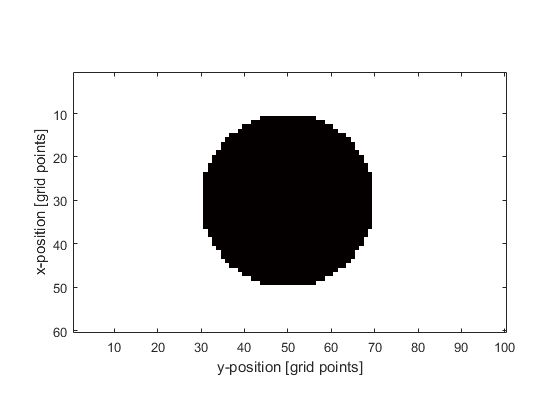# makeDisc

Create a binary map of a filled disc within a 2D grid.

## Syntax

```disc = makeDisc(Nx, Ny, cx, cy, radius)
disc = makeDisc(Nx, Ny, cx, cy, radius, plot_disc)
```

## Description

`makeDisc` creates a binary map of a filled disc within a two-dimensional grid (the disc position is denoted by 1's in the matrix with 0's elsewhere). A single grid point is taken as the disc centre thus the total diameter of the disc will always be an odd number of grid points. As the returned disc has a constant grid point radius, if used within a k-space grid where dx and dy are not equal, the disc will appear oval shaped. If part of the disc overlaps the grid edge, the rest of the disc will wrap to the grid edge on the opposite side. The plot that is displayed after calling `makeDisc(60, 100, 30, 50, 20, true);` is given below.## Inputs

 `Nx, Ny` size of the 2D grid [grid points] `cx, cy` centre of the disc [grid points] `radius` disc radius [grid points]

## Optional Inputs

 `plot_disc` Boolean controlling whether the disc is plotted using `imagesc` (default = false)

## Outputs

 `disc` 2D binary map of a filled disc

## Examples

`makeCircle`, `makeBall`